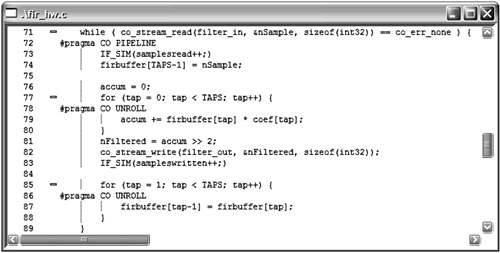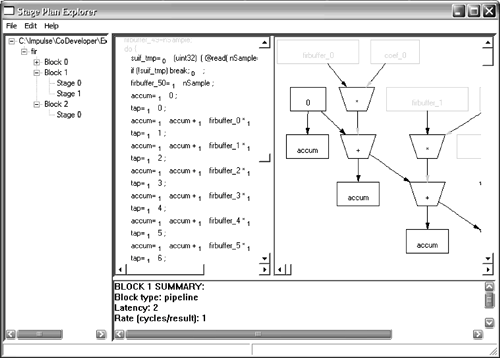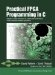# Section 7.3. Exploiting Instruction-Level Parallelism

### 7.3. Exploiting Instruction-Level Parallelism

The Impulse C tools include C and RTL optimizers that provide general C-language optimizations as well as a parallel optimizer (called Stage Master) that is specific to FPGA targets. When writing Impulse C processes, it is important to have a basic understanding of how C code is parallelized by these optimizers so you can achieve the best possible results in terms of execution speed and size of the resulting logic.

The optimizer works at the level of individual blocks of C code, such as are found within a process or loop body. For each block, the optimizer attempts to create the minimum number of instruction stages, using instruction pipelining where possible and by scheduling instructions that do not have conflicting data dependencies.

#### Instruction Scheduling

Scheduling includes three major activities: parallelism extraction, control inference, and register insertion. Parallelism extraction is critical for obtaining optimal hardware performance from an inherently sequential algorithm description. While coarse-grained parallelism is often better expressed at the system level and even through the actual system partitioning (for example, using two processors implies a level of parallelism), fine-grained parallelism is often difficult for a designer to code or even recognize for a complex algorithm. C-to-RTL compilers automatically perform comprehensive data dependency analysis to find and exploit parallelism in a design. This parallelism is exploited during scheduling of the design. The use of other, user-specified optimizations such as pipelining and loop unrolling may allow the creation of additional parallelism.

#### Pipeline Generation

If pipelining is enabled (via the PIPELINE pragma, which is added within the body of a loop and applies only to that loop), such identified stages occur in parallel. If no pipelining is possible, the stages are generated sequentially. Note that all statements within a stage are implemented in combinational logic operating within a single clock cycle.

There are three main opportunities for instruction-level parallelizing in C code:

1. Suboperations of a complex expression may be performed in parallel. A simple example of this might be an expression such as x = (a + b) * (c + d). In a traditional processor the two add operations would require two distinct instruction cycles to determine the sums prior to the multiply being performed. In a parallel implementation, the two sums could take place in the same instruction cycle, in parallel. This is possible because the two operations a + b and c + d have no timing-sensitive interdependencies.

2. Multiple statements in a fragment of straight-line code may be performed in parallel. For sequences of instructions in which there are no interdependencies, such as in the following statements:

` x = a + b; y = a + c; `

These two statements (and more such statements) can be implemented as parallel hardware structures, potentially requiring only a single clock cycle for processing.

3. Multiple iterations of a loop may be performed in parallel, using a technique called pipelining. Pipelining allows the next iteration of a loop to begin executing before completing the current iteration in parallel with the execution of the current iteration.

Examples of all three of these types are presented in this and subsequent chapters. First, however, let's review how software-to-hardware compilation is performed and how the compiler looks for the sort of parallelism just described.

#### Optimizer Operation

The basic operation of the Impulse optimizer (Stage Master) is to divide a C procedure into basic blocks and then assign each operation of each basic block to one or more stages. Figure 7-1 illustrates how one stage of the FIR filter example (presented in Chapters 5 and 6) represents multiple parallel operations.

##### Figure 7-1. During optimization, C statements are are divided into blocks and stages.In general, each stage is performed in a single clock cycle in hardware. The exception is that if a particular stage is waiting for data from an external source (such as a stream or memory), that stage may stall for one or more clock cycles until the data arrives. Stages are performed one at a time and the generated hardware controller determines what stage is being performed and which stage should be performed next.

The optimizations performed include four basic passes. The first pass (optional and controlled by the UNROLL pragma) is to unroll inner loops and replicate their code blocks to create parallel structures. Next, a scheduling pass determines the minimum number of stepsor stagespossible to implement each basic block of statements. During this pass, each operation in a basic block is assigned a stage. All operations assigned to a given stage execute in parallel. A third optimization pass attempts to balance the delays of the individual stages. For each loop being pipelined (controlled by the PIPELINE pragma), a final pass analyzes resource constraints and interiteration dependencies to determine when and how much of the next iteration can begin in parallel with current iteration.

#### Expression-Level Optimizations

The optimizer automatically parallelizes computations both within and across multiple expressions and statements. For example, in the following code:

` X = a + b + c; X = X << 2; Y = a - c; `

the optimizer assigns the two additions of the first statement, the shift operation of the second statement, and the subtract operation of the third statement to a single stage.

Note that if many operations were to be chained together in this way within a single stage, the maximum clock speed could be seriously reduced. This is because each stage is to be performed in a single cycle, regardless of the amount of combinational logic required to implement the described operation. By using the StageDelay option in Impulse C, the programmer can control this by specifying the maximum delay (measured as an estimated number of gate delays) that the optimizer will allow in a single stage.

#### Optimization Within Basic Blocks

A basic block is a contiguous block of straight-line code with no intervening control statements such as if, while, and so on. For example, if the previous example were this instead:

` if (odd) {     x = a + b + c;     x = x << 2; } y = a - c; `

the optimizer would generate one stage to perform (a + b + c) << 2 and a second stage to compute a - c.Practical FPGA Programming in C
ISBN: 0131543180
EAN: 2147483647
Year: 2005
Pages: 208